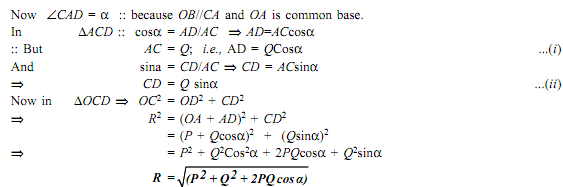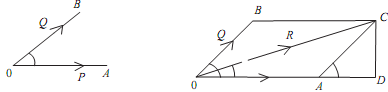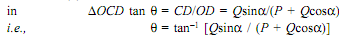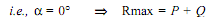The Eduladder is a community of students, teachers, and programmers just interested to make you pass any exams. So we help you to solve your academic and programming questions fast.
Watch related videos of your favorite subject.
Connect with students from different parts of the world.
See Our team
Wondering how we keep quality?
Got unsolved questions?

Civil-engineering-Common-for-all-semester-->View question

## State and prove parellelogram law of forces

Elements of civil engineering and engineering mechanics

Taged users:
rathinlz04

Likes:
Be first to like this question

Dislikes:
Be first to dislike this question

Prove the parallelogram law of forces:

Assume that the two forces and act at a point 'O' as shown in figure given below. The force can be represented in magnitude and direction by vector OA, While force is represented in magnitude and direction by vector OB, Angle between the two force is 'a'. The resultant can be denoted by vector OC as shown in the figure given below. Drop perpendicular from on OA.

Let,

P,= Forces whose resultant is needed to be found out.

θ= Angle that the resultant forces makes with one of the forces

α= Angle between the forces and QIt is the magnitude of resultant 'R'Direction ( θ ):Conditions

(i) Resultant R is max when two forces collinear and are in the same direction.(ii) Resultant R is min when two forces collinear but acting in the opposite direction.

That is  α = 1800    =>   Rmin = PQ

(iii) If a = 900, that is when the forces act at right angle, then

=   P2  + Q2

(iv) If the two forces are equal that is, when Q  =>R = 2P.cos(θ/2)

Likes:
Be first to like this answer

Dislikes:
Be first to dislike this answer

### Watch more videos from this user Here

Learn how to upload a video over here

Lets together make the web is a better place

We made eduladder by keeping the ideology of building a supermarket of all the educational material available under one roof. We are doing it with the help of individual contributors like you, interns and employees. So the resources you are looking for can be easily available and accessible also with the freedom of remix reuse and reshare our content under the terms of creative commons license with attribution required close.

You can also contribute to our vision of "Helping student to pass any exams" with these.